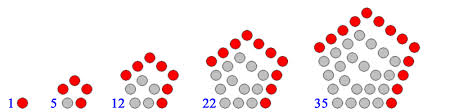# A problem in honour of solving 1000 problemsIs 1001 a pentagonal number?

Note: If yes, then enter the term number of 1001 in the sequence of pentagonal numbers as your answer. For example, the term number of 12 is 3, so you will enter the answer as 3; If no, then enter the answer as 1001.

Bonus question: How will you find whether a number is a pentagonal number or not? Is there any test?

×

Problem Loading...

Note Loading...

Set Loading...